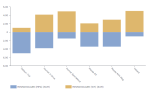# DCPY.MINMAXSCALER(feature_range_min, feature_range_max, column)

MinMaxScaler scales the data to a fixed range specified by the user. It basically shrinks the data to the specified range. When compared to the Standard Scaler, it works better if the distribution is not normal, or the standard deviation is very small. It is, however, very sensitive to outliers. It is an alternative to Z-score (Standard) normalization.

###### Parameters
• feature_range_min – Lower bound of desired range of transformed data, float (for example, 0).
• feature_range_max – Upper bound of desired range of transformed data, float (for example, 1).
• column – Dataset column or custom calculation.

Example: DCPY.MINMAXSCALER(0, 1, [Discount])

###### Input data
• A numeric column
• Rows that contain missing values are dropped before calculations
###### Result
• Numeric column with transformed values with same length as input column.
• Missing values are on the same indices like in input column.
###### Key usage points
• Use it when you need to compare variables on the same scale or specified custom range.
• Use it with not normal distributions, on data that has very small standard deviation without outliers.
###### Example

The following example shows how the car weight and fuel economy (mpg) are scaled to the range from 1 to 5 by using the following functions:

• DCPY.MINMAXSCALER(1, 5, [MPG])

• DCPY.MINMAXSCALER(1, 5, [WT])

The two scaled values are visualized in the Butterfly visualization.For the whole list of algorithms, see Data science built-in algorithms.